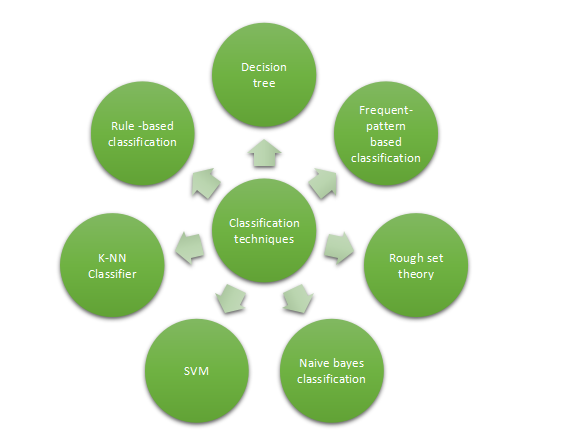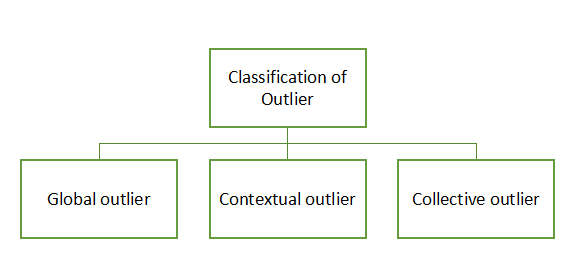Related Articles

# Classification-Based Approaches in Data Mining

• Difficulty Level : Medium
• Last Updated : 04 Aug, 2021

Classification is that the processing of finding a group of models (or functions) that describe and distinguish data classes or concepts, for the aim of having the ability to use the model to predict the category of objects whose class label is unknown. The determined model depends on the investigation of a set of training data information (i.e. data objects whose class label is known). The derived model could also be represented in various forms, like classification (if – then) rules, decision trees, and neural networks. Data Mining has a different type of classifier: A classification is a form of data analysis that extracts models describing important data classes. Such models are called Classifiers. For example, We can build a classification model for banks to categorize loan applications.

### A general approach to classification:

Classification is a two-step process involving,

Learning Step: It is a step where the Classification model is to be constructed. In this phase, training data are analyzed by a classification Algorithm.

Classification Step: it’s a step where the model is employed to predict class labels for given data. In this phase, test data are wont to estimate the accuracy of classification rules.

### Basic algorithms of classification:Decision Tree Induction:

• Decision Tree Induction is the learning of decision trees from class labeled training tuples.
• Given a tuple X, for which the association class label is unknown the attribute values of tuples are tested against the decision tree.
• A path that is traced from the root to the leaf node, which holds the class prediction for the tuple.
• These trees are then converted into Classification rules.
• Decision Trees are easier to interrupt are they no need any domain Knowledge

Naïve Bayesian Classification:

• They are Statistical Classifiers.
• They can predict class membership probabilities such as the probability that a given tuple belongs to a particular class.
• Naïve classifiers assume that the effect of an attribute value on a class is independent of values of other attributes.
• The mathematical formula for this classification is,where H be a hypothesis and p(H|X) is a probability that H holds the given evidence for the tuple X (Observed data)

p(X|H) is the  posterior probability of X conditioned on H

Rule-Based Classification:

• Rules are a good way of representing information or knowledge.
• A rule-based classifier uses a set of IF-THEN rules for Classification and is represented as• The IF part is called as Precondition and THEN part is called as rule consequent.
• This implies that only if the condition is met is the next(THEN) part will execute.

Now let’s see how to classify Outlier. A database may contain data objects that don’t suits the overall behavior or model of the info . These data objects are Outliers. The investigation of OUTLIER data is understood as OUTLIER MINING. An outlier could also be detected or classify using statistical tests which assume a distribution or probability model for the info , or using distance measures where objects having alittle fraction of “close” neighbors in space are considered outliers. Rather than utilizing factual or distance measures, deviation-based techniques distinguish exceptions/outliers by inspecting differences within the principle attributes of things during a group.

Outlier detection (also referred to as anomaly detection) is that the process of finding data objects with behaviors that are very different from expectations. Such objects are called outliers or anomalies. Outlier detection is vital in many applications additionally to fraud detection like medical aid , public safety and security, industry damage detection, image processing, sensor/video network surveillance, and intrusion detection.

In general, outliers are often classified into three categories, namely global outliers, contextual (or conditional) outliers, and collective outliers. Let’s examine each of these categories.Global Outliers: during a given data set, a knowledge object may be a global outlier if it deviates significantly from the remainder of the info set. Global outliers are sometimes called point anomalies and are the only sort of outliers. Most outlier detection methods are aimed toward finding global outliers.

Contextual Outliers: during a given data set, a knowledge object could also be a contextual outlier if it deviates significantly with regard to a specific context of the thing. Contextual outliers also are referred to as conditional outliers because they’re conditional on the chosen context. Therefore, in contextual outlier detection, the context possesses to be specified as a neighborhood of the matter definition. Unlike global outlier detection, in contextual outlier detection, whether a knowledge object is an outlier depends on not only the behavioral attributes but also the contextual attributes. Contextual outliers are a generalization of local outliers, a notion introduced in density-based outlier analysis approaches. An object during a data set may be a local outlier if its density significantly deviates from the local area during which it occurs.

Collective Outliers: during a given data set, a subset of knowledge objects forms a collective outlier if the objects as an entire deviate significantly from the whole data set. Importantly, the individual data objects might not be outliers. Unlike global or contextual outlier detection, in collective outlier detection, we’ve to think about not only the behavior of individual objects but also that of groups of objects. Therefore, to detect collective outliers, we’d like the background of the connection among data objects like distance or similarity measurements between objects.

Attention reader! Don’t stop learning now. Get hold of all the important CS Theory concepts for SDE interviews with the CS Theory Course at a student-friendly price and become industry ready.

My Personal Notes arrow_drop_up